# 吴恩达机器学习 - 推荐系统

## 笔记：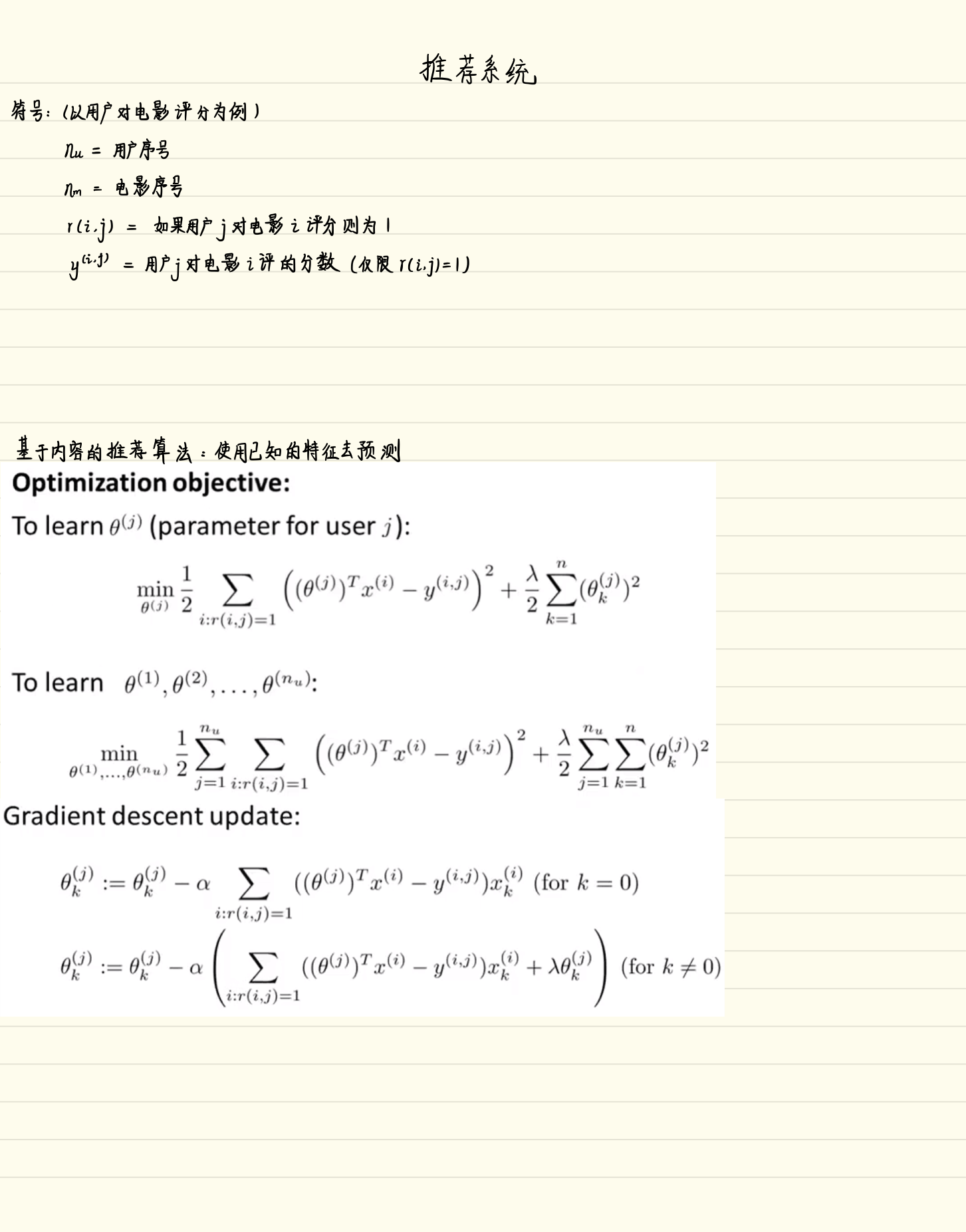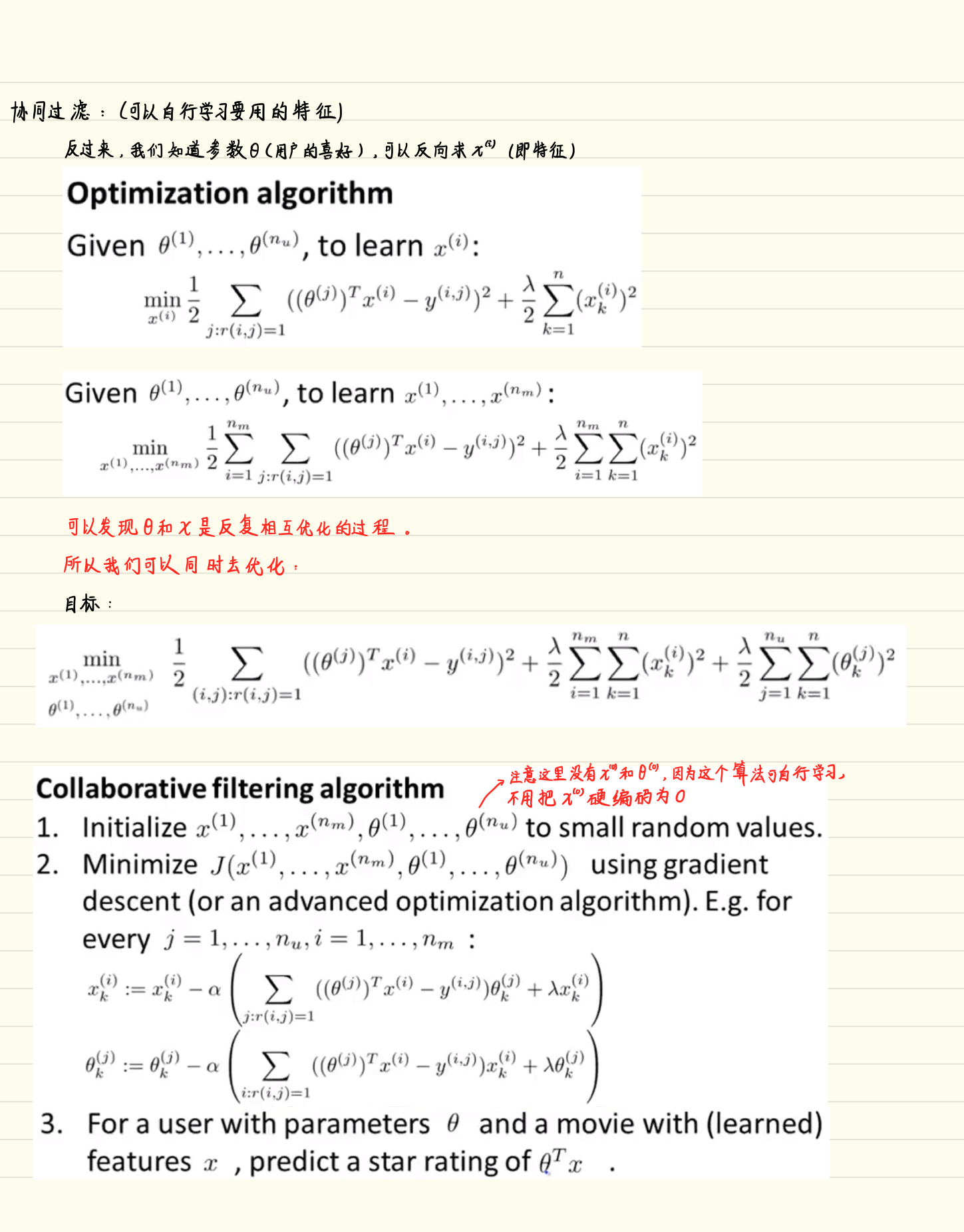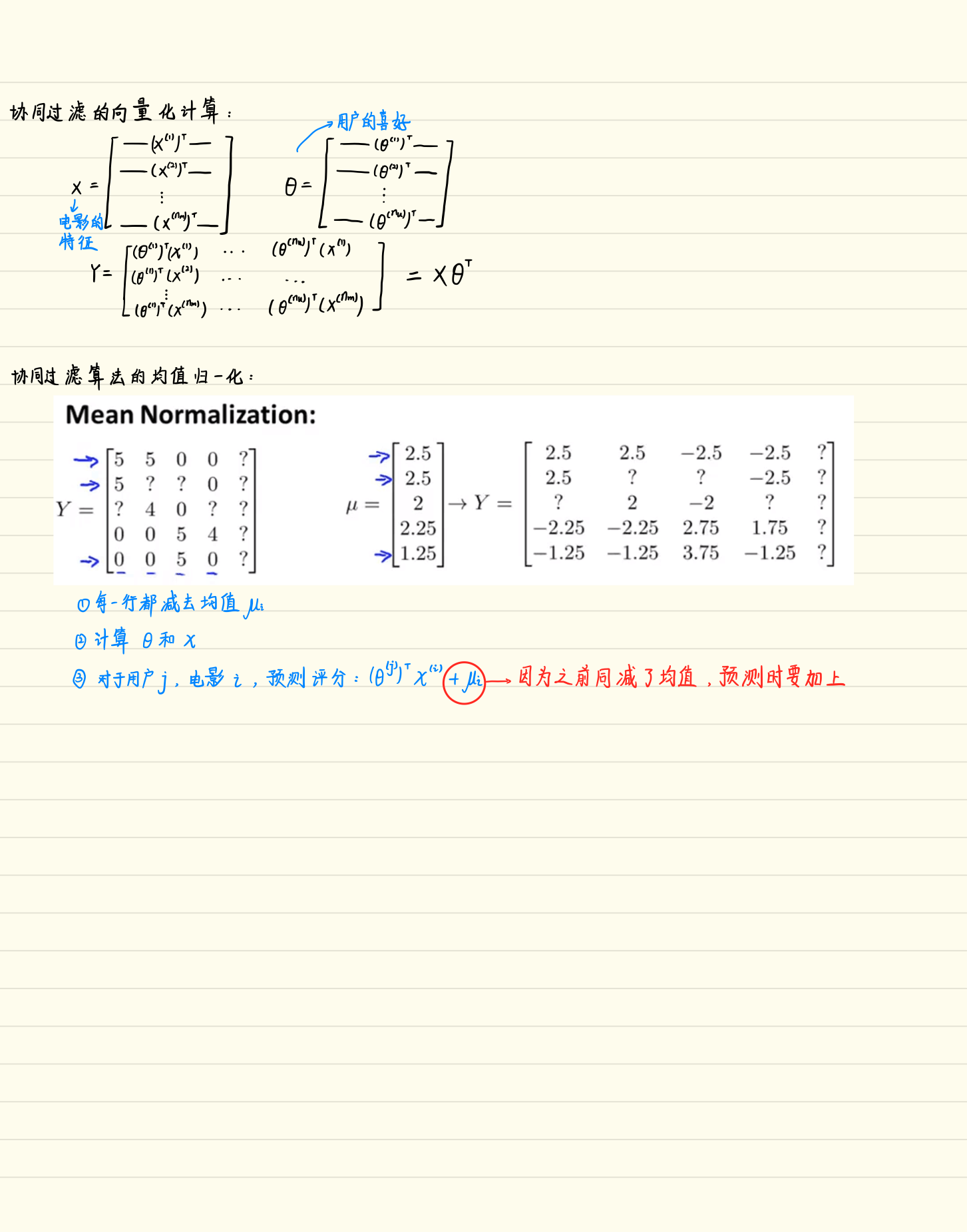## 每个算法最重要的莫过于代价函数了：

#### 公式：

###### 求代价：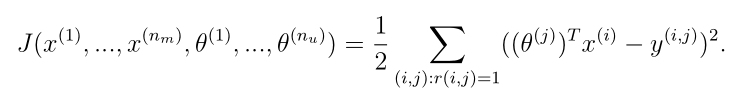###### 求梯度：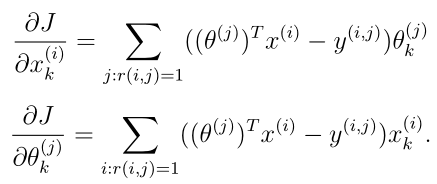#### Code（cofiCostFunc.m）：

function [J, grad] = cofiCostFunc(params, Y, R, num_users, num_movies, ...
num_features, lambda)
%COFICOSTFUNC Collaborative filtering cost function
%   [J, grad] = COFICOSTFUNC(params, Y, R, num_users, num_movies, ...
%   num_features, lambda) returns the cost and gradient for the
%   collaborative filtering problem.
%

% Unfold the U and W matrices from params
X = reshape(params(1:num_movies*num_features), num_movies, num_features);
Theta = reshape(params(num_movies*num_features+1:end), ...
num_users, num_features);

% You need to return the following values correctly
J = 0;

% ====================== YOUR CODE HERE ======================
% Instructions: Compute the cost function and gradient for collaborative
%               filtering. Concretely, you should first implement the cost
%               function (without regularization) and make sure it is
%               matches our costs. After that, you should implement the
%               gradient and use the checkCostFunction routine to check
%               that the gradient is correct. Finally, you should implement
%               regularization.
%
% Notes: X - num_movies  x num_features matrix of movie features
%        Theta - num_users  x num_features matrix of user features
%        Y - num_movies x num_users matrix of user ratings of movies
%        R - num_movies x num_users matrix, where R(i, j) = 1 if the
%            i-th movie was rated by the j-th user
%
% You should set the following variables correctly:
%
%        X_grad - num_movies x num_features matrix, containing the
%                 partial derivatives w.r.t. to each element of X
%        Theta_grad - num_users x num_features matrix, containing the
%                     partial derivatives w.r.t. to each element of Theta
%

%求代价
J = sum(sum((R.*(X*Theta')-Y).^2))/2.0 + ...
lambda/2.0*sum(sum(Theta.^2)) + lambda/2.0*sum(sum(X.^2));

%求梯度（这一步的向量化计算不好理解，调试看维度才知道谁乘谁）
X_grad = (R.*(X*Theta')-Y)*Theta + lambda.*X;
Theta_grad = (R.*(X*Theta')-Y)'*X + lambda.*Theta;

% =============================================================

end